# PSAT Math : How to find the solution to an inequality with subtraction

## Example Questions

### Example Question #1 : How To Find The Solution To An Inequality With Subtraction

|12x + 3y| < 15

What is the range of values for y, expressed in terms of x?

y < 5 – 4x

5 + 4x < y < 5 – 4x

y > 15 – 12x

–5 – 4x < y < 5 – 4x

5 – 4x < y < 5 + 4x

–5 – 4x < y < 5 – 4x

Explanation:

Recall that with absolute values and "less than" inequalities, we have to hold the following:

12x + 3y < 15

AND

12x + 3y > –15

Otherwise written, this is:

–15 < 12x + 3y < 15

In this form, we can solve for y. First, we have to subtract x from all 3 parts of the inequality:

–15 – 12x < 3y < 15 – 12x

Now, we have to divide each element by 3:

(–15 – 12x)/3 < y < (15 – 12x)/3

This simplifies to:

–5 – 4x < y < 5 – 4x

### Example Question #41 : Equations / Inequalities

|4x + 14| > 30

What is a possible valid value of x?

1

4

–11

7

–3

7

Explanation:

This inequality could be rewritten as:

4x + 14 > 30  OR 4x + 14 < –30

Solve each for x:

4x + 14 > 30; 4x > 16; x > 4

4x + 14 < –30; 4x < –44; x < –11

Therefore, anything between –11 and 4 (inclusive) will not work. Hence, the answer is 7.

### Example Question #3 : How To Find The Solution To An Inequality With Subtraction

Given the inequality,  |2x – 2|  >  20,

what is a possible value for x?

–8

11

10

0

–10

–10

Explanation:

For this problem, we must take into account the absolute value.

First, we solve for 2x – 2 > 20.  But we must also solve for 2x – 2 < –20 (please notice that we negate 20 and we also flip the inequality sign).

First step:

2x – 2 > 20

2x > 22

x > 11

Second step:

2x – 2 < –20

2x < –18

x < –9

Therefore, x > 11 and x < –9.

A possible value for x would be –10 since that is less than –9.

Note: the value 11 would not be a possible value for x because the inequality sign given does not include an equal sign.

### Example Question #4 : How To Find The Solution To An Inequality With Subtraction

Solve for.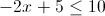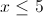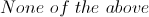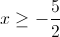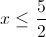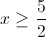Explanation:

Move +5 using subtraction rule which will give you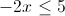Divide both sides by 2 (using division rule) and you will get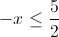which is the same as### Example Question #6 : How To Find The Solution To An Inequality With Subtraction

If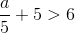, which of the following MUST be true?

I.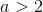II.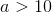III.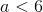I, II, and III

I and II only

III only

I only

II only

I only

Explanation:

Subtract 5 from both sides of the inequality: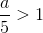Multiply both sides by 5:Therefore only I must be true.

### Example Question #1 : Inequalities

Which of the following is equivalent to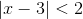?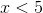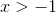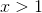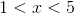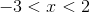Explanation:

Solve for both x – 3 < 2 and –(x – 3) < 2.

x – 3 < 2 and –x + 3 < 2

x < 2 + 3 and –x < 2 – 3

x < 5 and –x < –1

x < 5 and x > 1

The results are x < 5 and x > 1.

Combine the two inequalities to get 1 < x < 5

### Example Question #1 : Inequalities

Which of the following is a possible set of solutions to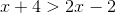?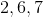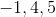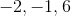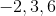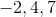Explanation:

Manipulate the inequality untilis on a side by itself: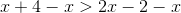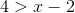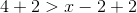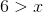For this equation,must be less than 6. Find the answer choice with values all less than 6. In this case, it will be -1, 4, and 5.

### Example Question #11 : Inequalities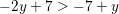Given the inequality above, which of the following MUST be true?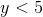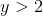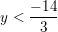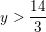Explanation:Subtractfrom both sides: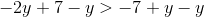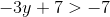Subtract 7 from both sides: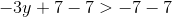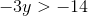Divide both sides by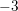: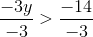Remember to switch the inequality when dividing by a negative number: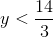Since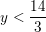is not an answer, we must find an answer that, at the very least, does not contradict the fact thatis less than (approximately) 4.67.  Since any number that is less than 4.67 is also less than any number that is bigger than 4.67, we can be sure thatis less than 5.

### Example Question #132 : Algebra

A factory packs cereal boxes. Before sealing each box, a machine weighs it to ensure that it is no lighter than 356 grams and no heavier than 364 grams. If the box holds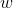grams of cereal, which inequality represents all allowable values of?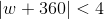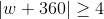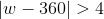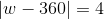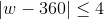Explanation:

The median weight of a box of cereal is 360 grams. This should be an allowable value of w. Substituting 360 for w into each answer choice, the only true results are: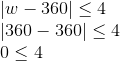and: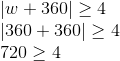Notice that any positive value for w satisfies the second inequality above. Since w must be between 356 and 364, the first inequality above is the only reasonable choice.